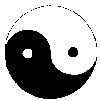Mathematics and Informatics in Old Symbols:
Tai Chi Symbol and Hexagrams from the I Ging

Klaus-D. Graf
Institut für Informatik, Freie Universität Berlin
D-14195 Berlin, Germany

Abstract

The paper describes how the  geometric  properties  of  the  Tai  Chi  Symbol  can  be  exploited
in geometry education to intensify the studies on circles  and  to  introduce  some  views  which
are different from the standard views on  elementary  notions  such  as  diameter,  splitting  of
areas or straightening, of arcs. Also, due  to  the  philosophical  background  of  the  Tai  Chi
Symbol, interdisciplinary aspects as  well  as  intercultural  aspects  can  be  integrated  into
mathematics education. Similar reasons can be given  for  teaching  the  hexagrams  from  the  I
Ging, in computer science education. They reveal very interesting aspects of binary coding.

Keywords

Tai Chi Symbol. Yin Yang, geometry  of  the  circle,  circular  inversion.  straightification  of
circular arcs. hexagrams, binary codinly.

1. The Tai Chi Symbol

The  Buddhist  Tai  Chi  Symbol  (TC  Symbol),  also  called  Yin  Yang  Symbol,  has  a very
strong graphical appeal. It is very often used  for  decoration.  In  modem  representations  the
separation line is formed bv two semicircles, each having  half  the  radius  of  the  enclosing
circle. (fig. 1). In the following we will call this line a yin yang diameter  (yy  diameter).  Old
representations are based on different construction modes as shown in figures 2 and 3.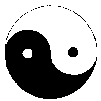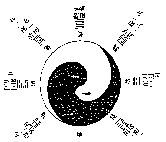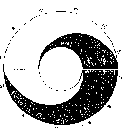Fig.1                                    Fig.2                                  Fig.3

In the  modern  as  well  as  in  the  old  symbols  you  can  discover  mathematical  symmetries
which possibly give a formal  background  for  the  aesthetic  appeal  of  the  symbol.  The  two
areas created bv the separation line. e.a., are congruent  and  they  have  a  central  symmetry
related to the center of the circle. Their circumferences are also equal to the perimeter of  the
enclosing circle.

2. Different philosophies of splitting a circle

The problem of splitting the area of the circle into equal parts has  been  handled  in  art  and
mathematics since ancient times. I found solutions of  a  very  high  artistic  standard  in  a
temple at the old city of Nara, Japan  (fig.  9)  and  on  a  door  in  the  old  German  castle
Wartburg (early 15th century, fig.  10).  There  are  obvious  similarities  in  the  yin  yang
solution  of  the  problem  both  in  Japan  and  Germany,  and  there  are  generalizations  of
splittings with three, four and more areas. In European decorative arts the  shape  of  a  fish-
bladder is taken as a basic element of symmetry in the circle (fig. 11).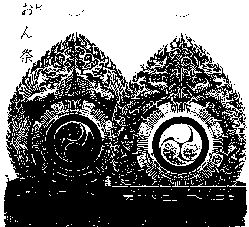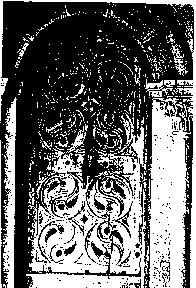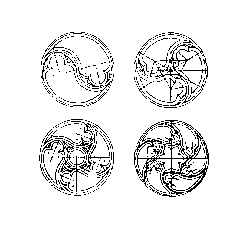Fig.9                                        Fig.10                                        Fig.11

From the point of view of pure mathematics  or  geometry,  the  most  simple  way  of  splitting
a circle into two equal parts is by using a straight diameter (see fig. 4). To get n  equal  areas
you use n/2 diameters, if n is even. For an odd n the splitting can be done by  using  radii.  It
is well known, however, that  ruler  and  compass  can  help  in  this  construction  for  some  n
only. n has to be a product of powers of 2 and Format primes such as  3,  4,  5,  6,  8,  10,  15,
16, ... 65537. The same rules apply if  yy  diameters  are  used  instead  of  straight  diameters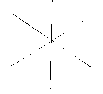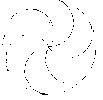Fig.4                           Fig.5                          Fig.6

Using, straight lines seems to express more  efficiency,  using  semicircles  reveals  more  har-
mony in the  splitting.  The  mode  which  is  chosen  possibly  reveals  an  Eastern  or  Western
philosophical attitude. Figure  12  shows  how  this  idea  is  underlined  by  a  symbol  on  the
cover of a book about the three main philosophical streams in China, India and Europe.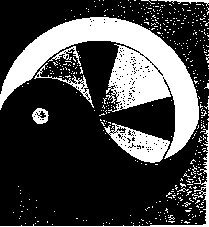Fig.12

3. Geometry of the circle in class - yy diameters

When  teaching  about  the  circle  in  geometry  education  in  schools,  its  interactions  with
straight lines or sections of a line  play  the  most  important  role.  You  look  at  diameters,
chords, secants, tangents etc. Interactions with other circles or circular arcs are studied  later
and less intensively. At the same time splitting of the area of the circle  are  constructed  and
discussed using straight lines.

Certainly one reason for this is the importance of this interaction for the solution of  practical
problems  related  to  areas  and  circumferences,  for  example.  From  a  systematic  point   of
view the idea is close, however, to examining the interactions of  circular  arcs  early  as  well
and to finding out mathematical relations and properties.

The Pythagorean number PAI is a  symbol  for  the  very  special  relation  between  circular  arcs
and straight line sections. PAI describes the metric relation between the diameter d of a  circle
and its perimeter u, u = d*PAI. At  the  same  time,  for  the  area  f  of  the  circle  we  have
f = (1/4)*d*d*PAI.  The  mathematical  nature  of  PAI forbids  the  construction  of  a  straight
section with a length equal to the perimeter of a circle using only ruler and compass.  as  well
as the "squaring of the circle".

These relations can be described in a different way using the yy diameter of a  circle.  In  its
modern representation this splitting, line of the  TC  symbol  is  composed  of  two  semicircles.
and it has some properties which are similar to those of the  classical  straight  diameter.  The
line runs through  the  center  of  the  circle,  its  endpoints  have  maximum  distance  on  the
perimeter of the circle and it has a central symmetry related to the center. The  line  does  not.
of course. generate an axial symmetry like the straight diameter.

It is obvious that the length of a yy diameter equals half  of  the  perimeter  of  its  circle.
Through rotation around the center we can get such yy diameters infinitely.

Naming the radius r, we have these formulae, avoiding PAI:

u = 2*t                f = r*t.

These formulae are not really "practical", since t, unlike r, is  not  measurable  with  a  metric
ruler, but they contain something of the harmony  which  the  splitting  of  a  circle  by  a  yy
diameter shows,  in  comparison  with  the  coolness  of  a  straight  section  with  a  standard
diameter.

Figure 7 shows that there are  more  lines  which  are  composed  of  two  semicircles  and  which
split a circle in a wave similar to the yy diameters.  Their  endpoints  halve  the  perimeter  and
their length equals t (=r*PAI). The diameters of  the  two  semicircles  are  d'  and  d-d',  where
0<d'<d. These "pseudo yy diameters" split the area r*t of the circle into two areas (r-r')*t
and r'*t, where r'=d'/2. Of course  if  r'=r/2  we  get  the  yy  diameter.  The  circumference  of
the two areas is the same as the perimeter of the surrounding circle.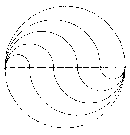Fig.7

So we see that t (=r*PAI) is not just a term or  half  of  the  perimeter,  but  characterizes  many
circle splitting, sections which are composed of semicircles.

Choosing a sequence r' = r/n (with n natural > 1), r" = 2*r/n, etc. you  get  a  splitting  of  the
area of a circle with radius r in (n+l) equal  areas  as  shown  in  figure  7.  This  method  goes
back to L. Collatz. This is easily seen, since if fi = i*r/n*t and f(i+1) =  (i+1)*r/n*t  are  two
of the areas generated with ri = i*r/n and r(i+l) = (i+l)*r/n, their difference is r*t/n.

This method of splitting the circle into n equal parts can  be  realized  with  ruler  and  compass
for any natural n>l. So  the  pseudo  yy  diameters  are  a  better  means  to  solve  the  problem
than standard diameters. On the other hand, we  must  admit  that  straight  diameters  are  easier
to construct for practical purposes.

Iterating the method of splitting the diameter d of a circle to get pseudo yy  diameters  brings
you to partitions rl+r2+...+rn = r which also generate pseudo  yy   diameters   of   length
t = (r*PAI). Putting- ri=r/n for all 1, with the  resulting  pseudo  yy  diameter  you  can  approach
the straight diameter as closely as you  want  by  just  choosing  n  large  enough.  The  differ-
ence in length is always t - d or PAI*r-2*r, however (figure 8).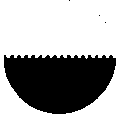Fig.8

Another interesting generalization of the idea of the yy  diameter  starts  with  intersection  a
circle with a straight line and studying the arc and  secant  section  thus  generated.  There  is
always a line similar to a yy diameter which has equal length with the arc.

4. Extension from a circle to the plane

Putting the Tai Chi Symbol on the unit  circle  of  a  complex  plane  and  applying  a  reciproque
complex mapplg of type z ->  1/z  you  extend  the  mathematical  idea  and  properties  of  this
symbol to the whole  plane  (figure  13).  The  image  of  a  Collatz  splitting  is  particularly
fascinating (figure 16). If  you  change  the  semicircles  from  the  yy  diameters  to  different
arcs, you get very interesting separation lines for the plane which have a strong graphical  or
even artistic appeal (figures 14 and 15).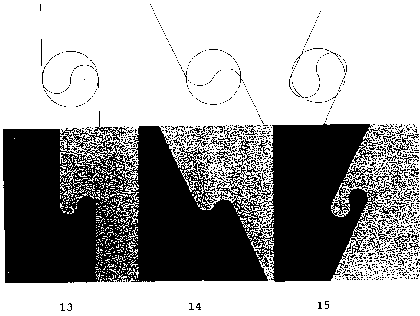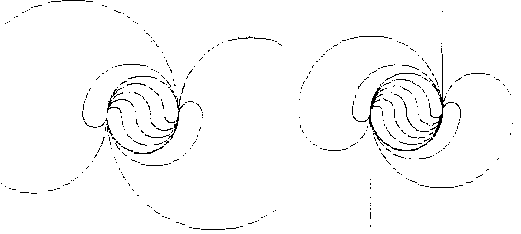Fig.16

5. "Straightening" of the yin yang diameter and of the perimeter

Euclidean geometry knows many ways of  approximating  the  length  of  a  perimeter  by  a
section of a straight line,  or  rather  a  sequence  of  such  sections  as  Given  by  a  regular
polygon.  Looking  at  Collatz'  figure  and  its  image  under   circular   inversion,   you   can
discover a method which gives  an  impression  of  how  a  semicircle  is  really  bent  towards  a
straight line. To discuss this method we do not need  the  background  of  the  Collatz  splitting
and its circular inversion.

We start with a TC symbol in a unit circle  with  radius  1.  Then  t=u/2=PAI.  Next  we  imbed  the
unit circle in a circle with radius 2 and perimeter 4*PAI as  shown  in  figure  17.  Now  t  equals
one fourth of this perimeter. Next we imbed in a circle with radius  4  and  t  equals  one  eighth
of its perimeter. Iterating this procedure we  get  a  sequence  of  circular  arcs  of  length  t.
which are getting "straighter and straighter". 1/4, 1/8, 1/16 etc. of a  circle's  perimeter  can
be constructed easily with ruler  and  compass;  so  we  have  a geometrically  effective  way  of
finding an arc of length t finally with the chord related to it coming close enough to t. This
is the "straightening" of the yy diameter we started with and it is half of the  "straightening"
of the surrounding circle.

Mathematically  this  method  corresponds  to  the  method  of   Archimedes,   who   approximates
the perimeter of a circle with regular polygons with  numbers  4,  8,  16,  32,  ...  or  6,  12,
24, ... of vertices.

6. Hexagrams from the I Ging

The hexagrams  from  the  I  Ging  became  public  in  Europe  through  an  exchange  of  letters
between G. W. Leibniz and  the  Jesuit  missionary  J.  Bouvet,  who  stayed  in  Beijing  around
1700 (figure 18). Leibniz was mostly  interested  in  the  interpretation  of  the  hexagrams  as
binary numbers. From the point of  view  of  modern  informatics  it  is  quite  clear,  however,
that the creators of the  hexagrams  more  than  5,000  years  ago  were  developing  a  binary
coding system. The purpose was to describe and classify all phenomena of the universe
growing out of Tai Chi, the universal power, by the interaction of yin and yang.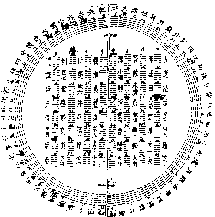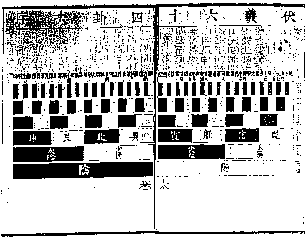Fig.18                                                    Fig.19

The system should also  describe  the  changes  between  the  phenomena,  such  as  from  winter
to spring to summer to fall and so on. This  is  one  reason  why  they  selected  orderings  for
the hexagrams which are different from  the  numerical  ordering.  Since  the  9th  century  A.D.
or earlier,  the  Chinese  have  known  the  ordering  following  the  binary  number  system.
Again, for them the meaning was different.  The ordering arises from a binary coding tree
(figure 19) of depth 6, which "by accident" yields the binary numbers  from  0  to  63  in  their
right order.

Another ordering clearly has combinatorial  character.  It  starts  with  one  hexagram  without
yins (0 yin), followed by six hexagrams with  one  yin,  15  with  two  yins,  20  with  3  yins
etc.  Research  in  mathematical  education  at  Rutgers  State  University  in  the  USA   (Bob
Davis) has shown that many primary  school  children  intuitively  choose  this   way   of
ordering when the following problem  is  put  to  them:  You  have  red  and  blue  cubes.
Construct all different towers with 3 (or 4 or 5) cubes.

References
  Graf,  K.D.:  Powerful   Means   in   Mathematics   and   Computer   Science   Education:
Mathematical, Logical, Mechanical  and  other  Roots  of  Computer  Science  in  History.
Jounal of  the  Cultural  History  of  Mathematics,  1,  Mathematics  Education  Society  of
Japan, 1991
  Needham,  J.:  Science  and  Civilization  in  China,  Vol.  I-IV,  1+2.   Cambridge   1954-
1965
  Zacher,  H.J.:  Die  Hauptschriften  zur  Dyadik  von  G.W.  Leibniz.  Frankfurt   am   Main
1973
  Mall,  Ram  A.  and  Hiilsmann:  Die  drei  Geburtsorte  der  Philosophic.  Bouvier-VerIaLy.
Bonn 1989Communication with the author:
Institut für Informatik
Takustr. 9
D-14195 Berlin
Germany
FAX: ++49-30-838-75-109     e-mai: graf@inf.fu-berlin.de